# How to Calculate CAGR in Excel

Compound annual growth rate, or CAGR, is your rate of return on an investment over a period of time.

Calculating CAGR by hand is a rather complex process, so below we will see how you can quickly calculate CAGR in Excel.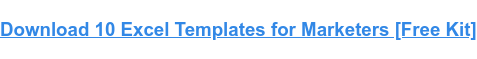## CAGR Excel Formula

The formula for calculating CAGR in Excel is:

=(Final value/Initial value) ^ (1/Number of years) – 1

The equation uses three different values:

• Final value, which is the amount of money you will have after the period is up.
• Starting valuewhich is the amount of money you started with.
• Number of yearswhich is the total number of years that have passed.

Below, we’ll walk through an example of calculating the CAGR for a five-year period in Excel using the sample dataset below: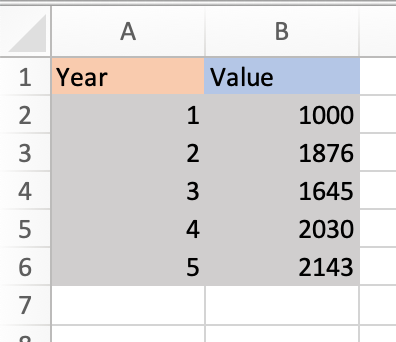1. Identify the numbers you will use in your equation. Using the example data above,

• The final value is 2143 (in cell B6).
• The starting value is 1000 (in cell B2).
• The number of years is 5 (in cell A6).

2. Enter your values ​​into the formula.

Excel offers many shortcuts, so you can simply enter the cell numbers containing each of your values ​​in the equation. Using the sample data above, the equation would be

= (B6 / B2) ^ (1 / A6) -1

This is what it looks like in my excel sheet: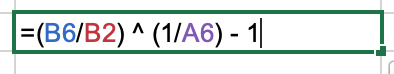Note that the equation changes color to match the cells you are using, so you can look back and check that your entries are correct before running the equation.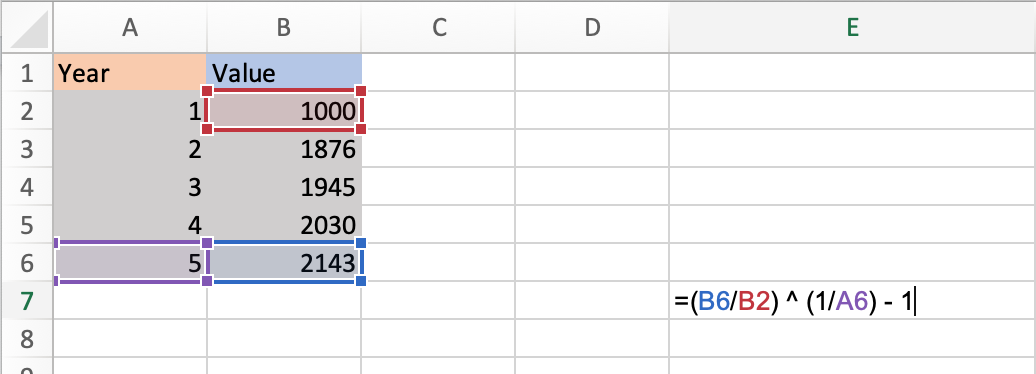You can also enter actual values ​​into the formula instead of cell numbers. The equation would then look like this:

=(2143/1000) ^ (1/5) – 1

3. Once you have entered your values, click Enter and run the equation. Your result will appear in the cell containing the equation as shown in the image below.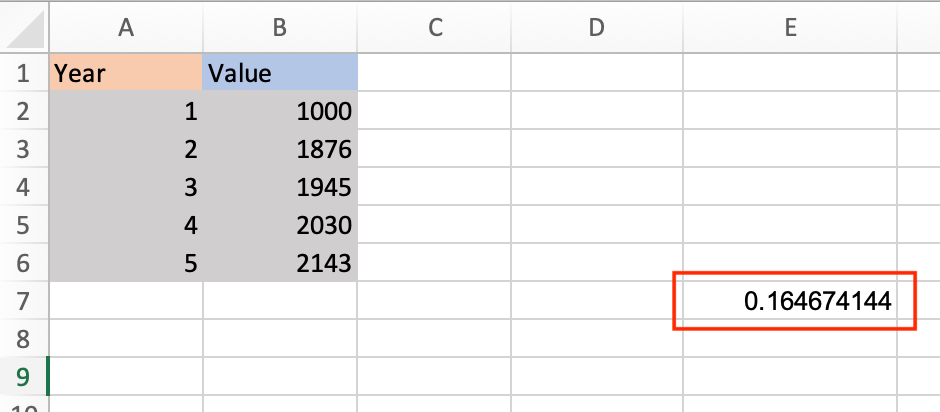### CAGR formula in Excel as a percentage

Your default result will be displayed as a decimal. To display it as a percentage, right click on the cell your result is in, select Format cells so what Percentage in the dialog box.

Your result will be converted to a percentage as shown in the image below.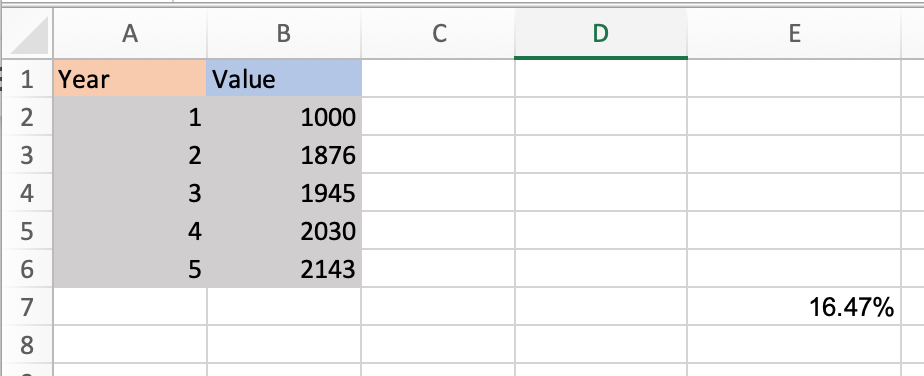Now let’s walk through a shortcut to calculate CAGR in Excel using the Rate function.

## How to calculate CAGR using the RATE function

The RATE function helps you calculate the interest rate of an investment over a given period. The CAGR calculation formula is as follows:

=RATE(nper,, pv, fv)

• nper is the total number of periods in the period you are measuring. Since you’re calculating the annual growth rate, that would be 12.
• PV is the current value of your investment (must always be represented by a negative)
• fv is the future value.

Note that the standard RATE equation includes more variables, but you only need the three above to calculate your CAGR.

Let’s run an equation using the example table below where nperi is 12, pv is 100 and fv is 500.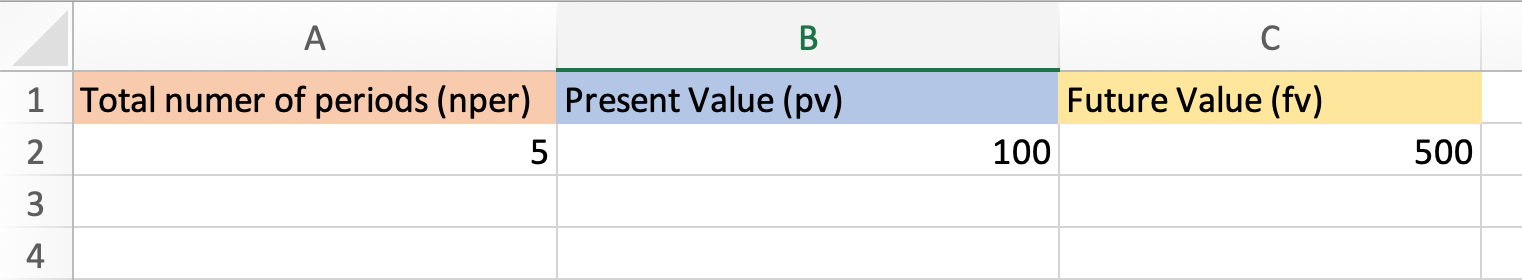1. In your sheet, select the cell in which you want to contain your CAGR. I selected cell B5.

2. Enter the formula RATE and enter your numbers. Note that you always must express your current value in negative form, otherwise you will receive an error message.

This is what my formula looks like.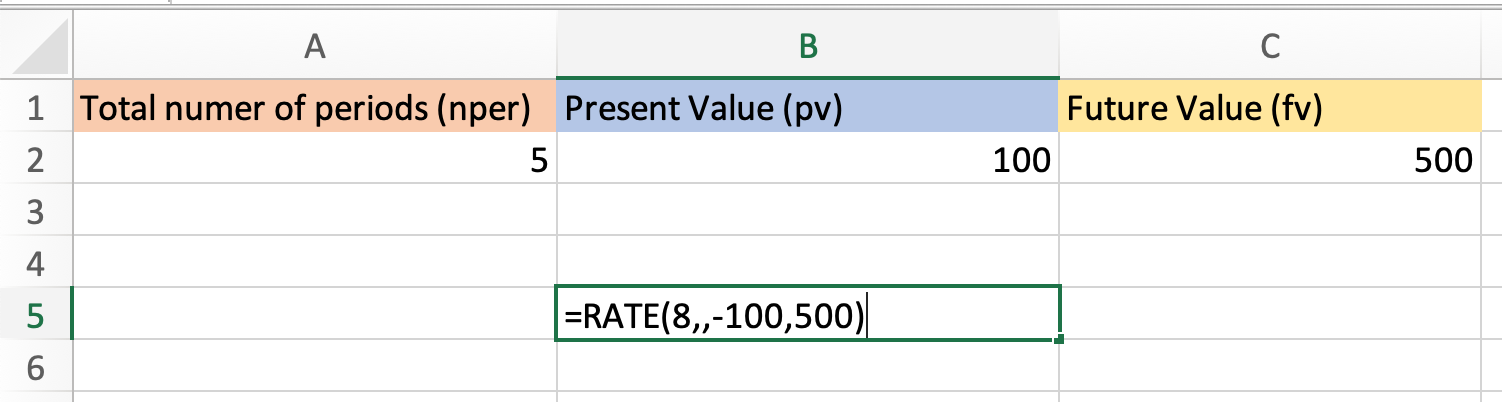Note that you can also just enter the cell numbers where your values ​​are. With my example table, the formula would look like this:

=RATE(A2,,-B2,C2)

3. Click Enter and run your equation. Using the sample data, my CAGR is 14%.

Now you know how to quickly and easily calculate your CAGR in Excel, no manual calculations required.